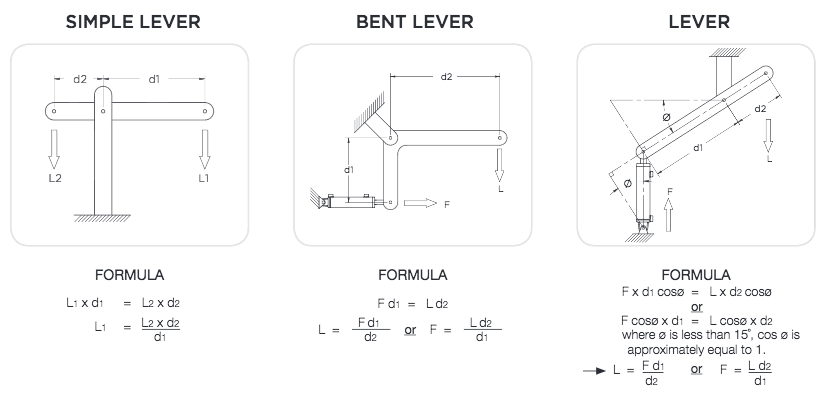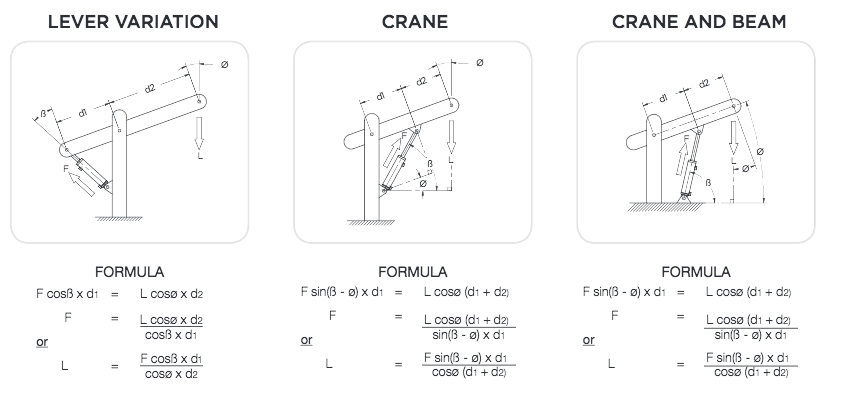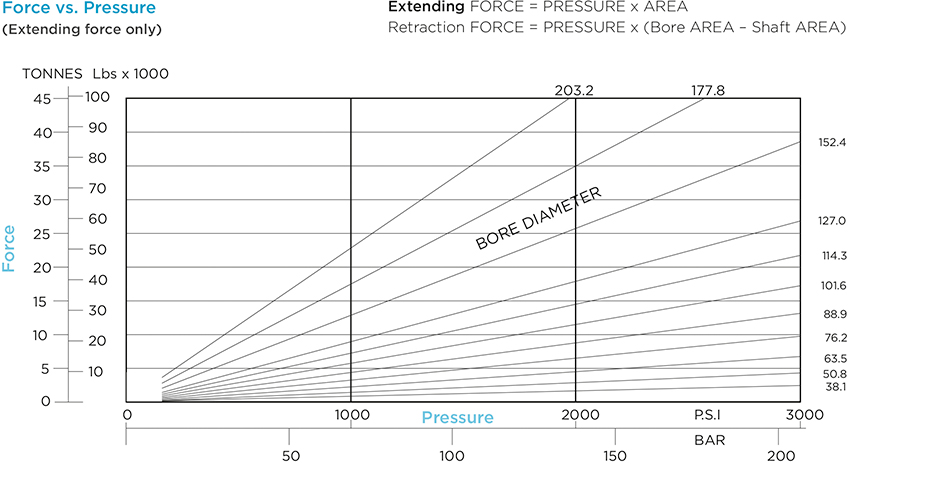Innovation | Quality | Delivery

# Design Theory

## Principles of moments#### Law of Moments: All moments in one direction equal all moments in the opposite direction

Moments = Force x Distance
but                 the direction of the force must be at right angles to the measurement of distance
therefore    Moment = Force x Distance at right angles to force
or                  Moment = Distance x component of Force operating at right angles to distance## Why shafts bend in hydraulic cylinders

Shafts in tension will not bend. Shafts in compression will bend if subjected to too much force. Excessive force may come from either too much pressure, or excessive mechanical loads applied to the shaft. For short shafts in compression, the limiting load is determined by the yield point of the material. For long shafts, the limiting load is a function of length and the mounting method.

## Calculate the shaft diameter needed

The method of supporting the ends of the shaft makes a considerable difference to the maximum load permitted. This difference is expressed by a constant called the “fixity factor” ( ƒƒ ). To calculate the maximum permitted extended length ( E ) for a given application and shaft diameter, multiply the “apparent length” ( L ) by the “fixity factor”. Select the appropriate "fixity factor" ( ƒƒ ) from the application guide below. ( L ) can be obtained from Euler’s formula.#### EULER’S FORMULA

For steel to En8 or AISI 1045, and a safety factor of 2.2 (i.e. a maximum permissible stress of 240 MPa), the formula is:## FIXITY FACTOR CHART

FOR RIGIDLY MOUNTED CYLINDERS: Fixity Factor                                     FOR PIN MOUNTED CYLINDERS: Fixity Factor## Theoretical Performance